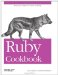# Representing Rational Numbers

Problem

You want to precisely represent a rational number like 2/3, one that has no finite decimal expansion.

Solution

Use a Rational object; it represents a rational number as an integer numerator and denominator.

```	float = 2.0/3.0 # => 0.666666666666667
float * 100 # => 66.6666666666667
float * 100 / 42 # => 1.58730158730159

require 'rational'
rational = Rational(2, 3) # => Rational(2, 3)
rational.to_f # => 0.666666666666667
rational * 100 # => Rational(200, 3)
rational * 100 / 42 # => Rational(100, 63)
```

Discussion

Rational objects can store numbers that can't be represented in any other form, and arithmetic on Rational objects is completely precise.

Since the numerator and denominator of a Rational can be Bignums, a Rational object can also represent numbers larger and smaller than those you can represent in floating-point. But math on BigDecimal objects is faster than on Rationals. BigDecimal objects are also usually easier to work with than Rationals, because most of us think of numbers in terms of their decimal expansions.

You should only use Rational objects when you need to represent rational numbers with perfect accuracy. When you do, be sure to use only Rationals, Fixnums, and Bignums in your calculations. Don't use any BigDecimals or floating-point numbers: arithmetic operations between a Rational and those types will return floating-point numbers, and you'll have lost precision forever.

```	10 + Rational(2,3) # => Rational(32, 3)
require 'bigdecimal'
BigDecimal('10') + Rational(2,3) # => 10.6666666666667
```

The methods in Ruby's Math module implement operations like square root, which usually give irrational results. When you pass a Rational number into one of the methods in the Math module, you get a floating-point number back:

```	Math::sqrt(Rational(2,3)) # => 0.816496580927726
Math::sqrt(Rational(25,1)) # => 5.0
Math::log10(Rational(100, 1)) # => 2.0
```

The mathn library adds miscellaneous functionality to Ruby's math functions. Among other things, it modifies the Math::sqrt method so that if you pass in a square number, you get a Fixnum back instead of a Float. This preserves precision whenever possible:

```	require 'mathn'
Math::sqrt(Rational(2,3)) # => 0.816496580927726
Math::sqrt(Rational(25,1)) # => 5
Math::sqrt(25) # => 5
Math::sqrt(25.0) # => 5.0
```

• The rfloat third-party library lets you use a Float-like interface that's actually backed by Rational (http://blade.nagaokaut.ac.jp/~sinara/ruby/rfloat/)
• RCR 320 proposes better interoperability between Rationals and floating-point numbers, including a Rational#approximate method that will let you convert the floating-point number 0.1 into Rational(1, 10) (http://www.rcrchive.net/rcr/show/320)Ruby Cookbook (Cookbooks (OReilly))
ISBN: 0596523696
EAN: 2147483647
Year: N/A
Pages: 399